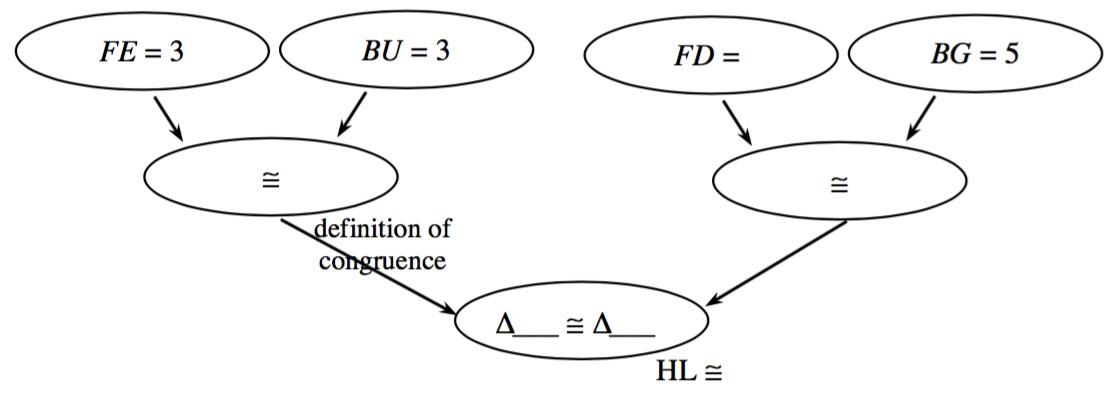Home > CC4 > Chapter 7 > Lesson 7.2.3 > Problem7-107

7-107.
1. For each pair of triangles below, determine whether or not the triangles are congruent. If they are congruent, show your reasoning in a flowchart. If they are not congruent, explain why not. Homework Help ✎

2.  a.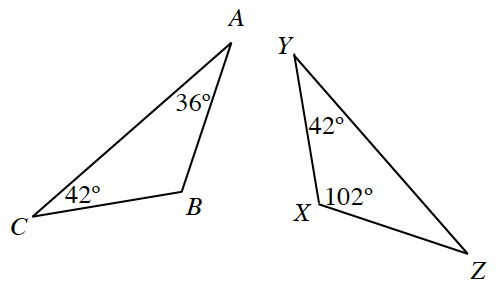b.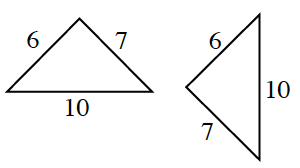c.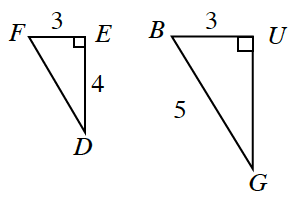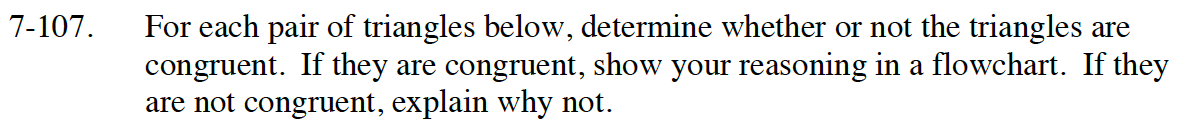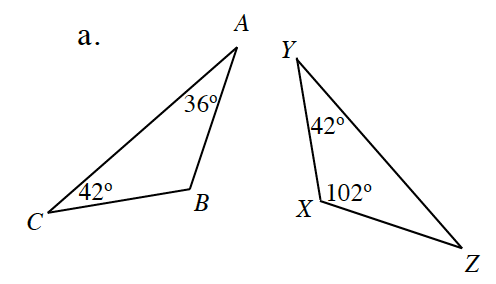Calculate the values of the missing angles. What do you notice?

Does AAA guarantee triangle congruence?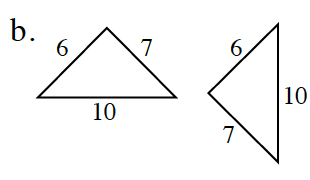Does SSS guarantee triangle congruence?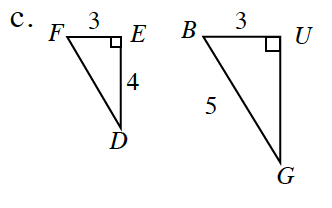These are both right triangles. Use the Pythagorean Theorem to calculate the lengths of the missing legs.

Complete the proof below.